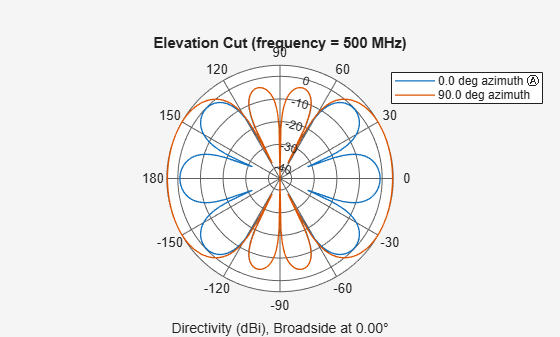# patternElevation

System object: phased.UCA
Package: phased

Plot UCA array directivity or pattern versus elevation

## Syntax

```patternElevation(sArray,FREQ) patternElevation(sArray,FREQ,AZ) patternElevation(sArray,FREQ,AZ,Name,Value) PAT = patternElevation(___) ```

## Description

`patternElevation(sArray,FREQ)` plots the 2-D array directivity pattern versus elevation (in dBi) for the array `sArray` at zero degrees azimuth angle. When `AZ` is a vector, multiple overlaid plots are created. The argument `FREQ` specifies the operating frequency.

The integration used when computing array directivity has a minimum sampling grid of 0.1 degrees. If an array pattern has a beamwidth smaller than this, the directivity value will be inaccurate.

`patternElevation(sArray,FREQ,AZ)`, in addition, plots the 2-D element directivity pattern versus elevation (in dBi) at the azimuth angle specified by `AZ`. When `AZ` is a vector, multiple overlaid plots are created.

`patternElevation(sArray,FREQ,AZ,Name,Value)` plots the array pattern with additional options specified by one or more `Name,Value` pair arguments.

`PAT = patternElevation(___)` returns the array pattern. `PAT` is a matrix whose entries represent the pattern at corresponding sampling points specified by the `'Elevation'` parameter and the `AZ` input argument.

## Input Arguments

expand all

Uniform circular array, specified as a `phased.UCA` System object.

Example: `sArray= phased.UCA;`

Frequency for computing directivity and pattern, specified as a positive scalar. Frequency units are in hertz.

• For an antenna or microphone element, `FREQ` must lie within the range of values specified by the `FrequencyRange` or the `FrequencyVector` property of the element. Otherwise, the element produces no response and the directivity is returned as `–Inf`. Most elements use the `FrequencyRange` property except for `phased.CustomAntennaElement` and `phased.CustomMicrophoneElement`, which use the `FrequencyVector` property.

• For an array of elements, `FREQ` must lie within the frequency range of the elements that make up the array. Otherwise, the array produces no response and the directivity is returned as `–Inf`.

Example: `1e8`

Data Types: `double`

Azimuth angles for computing sensor or array directivities and patterns, specified as a 1-by-N real-valued row vector where N is the number of desired azimuth directions. Angle units are in degrees. The azimuth angle must lie between –180° and 180°.

The azimuth angle is the angle between the x-axis and the projection of the direction vector onto the xy plane. This angle is positive when measured from the x-axis toward the y-axis.

Example: `[0,10,20]`

Data Types: `double`

### Name-Value Arguments

Specify optional pairs of arguments as `Name1=Value1,...,NameN=ValueN`, where `Name` is the argument name and `Value` is the corresponding value. Name-value arguments must appear after other arguments, but the order of the pairs does not matter.

Before R2021a, use commas to separate each name and value, and enclose `Name` in quotes.

Displayed pattern type, specified as the comma-separated pair consisting of `'Type'` and one of

• `'directivity'` — directivity pattern measured in dBi.

• `'efield'` — field pattern of the sensor or array. For acoustic sensors, the displayed pattern is for the scalar sound field.

• `'power'` — power pattern of the sensor or array defined as the square of the field pattern.

• `'powerdb'` — power pattern converted to dB.

Example: `'powerdb'`

Data Types: `char`

Signal propagation speed, specified as the comma-separated pair consisting of `'PropagationSpeed'` and a positive scalar in meters per second.

Example: `'PropagationSpeed',physconst('LightSpeed')`

Data Types: `double`

Array weights, specified as the comma-separated pair consisting of `'Weights'` and an M-by-1 complex-valued column vector. Array weights are applied to the elements of the array to produce array steering, tapering, or both. The dimension M is the number of elements in the array.

Note

Use complex weights to steer the array response toward different directions. You can create weights using the `phased.SteeringVector` System object or you can compute your own weights. In general, you apply Hermitian conjugation before using weights in any Phased Array System Toolbox™ function or System object such as `phased.Radiator` or `phased.Collector`. However, for the `directivity`, `pattern`, `patternAzimuth`, and `patternElevation` methods of any array System object use the steering vector without conjugation.

Example: `'Weights',ones(10,1)`

Data Types: `double`
Complex Number Support: Yes

Elevation angles, specified as the comma-separated pair consisting of `'Elevation'` and a 1-by-P real-valued row vector. Elevation angles define where the array pattern is calculated.

Example: `'Elevation',[-90:2:90]`

Data Types: `double`

Handle to the axes along which the array geometry is displayed specified as a scalar.

## Output Arguments

expand all

Array directivity or pattern, returned as an L-by-N real-valued matrix. The dimension L is the number of elevation angles determined by the `'Elevation'` name-value pair argument. The dimension N is the number of azimuth angles determined by the `AZ` argument.

## Examples

expand all

Create a 6-element UCA of short-dipole antenna elements. Design the array to have a radius of 0.5 meters. Plot an elevation cut of directivity at 0 and 90 degrees azimuth. Assume the operating frequency is 500 MHz.

```fc = 500e6; sCDant = phased.ShortDipoleAntennaElement('FrequencyRange',[100,900]*1e6); sUCA = phased.UCA('NumElements',6,'Radius',0.5,'Element',sCDant); patternElevation(sUCA,fc,[0 90])```You can plot a smaller range of elevation angles by setting the `Elevation` property.

`patternElevation(sUCA,fc,[0 45],'Elevation',[0:90])`